DraftKings Super Bowl Odds
+600
6 to 1Chiefs
11.7% implied probability

+650
6.5 to 1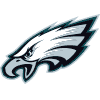Eagles
10.9% implied probability

+900
9 to 1Bills
8.2% implied probability

+1000
10 to 149ers
7.4% implied probability

+1100
11 to 1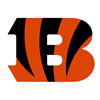Bengals
6.8% implied probability

+1400
14 to 1Cowboys
5.5% implied probability

+1800
18 to 1Jets
4.3% implied probability

+2000
20 to 1Ravens
3.9% implied probability

+2200
22 to 1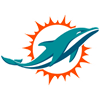Dolphins
3.6% implied probability

+2200
22 to 1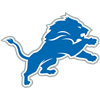Lions
3.6% implied probability

+2500
25 to 1Jaguars
3.2% implied probability

+2500
25 to 1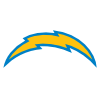Chargers
3.2% implied probability

+3000
30 to 1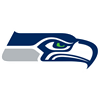Seahawks
2.6% implied probability

+3500
35 to 1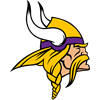Vikings
2.3% implied probability

+3500
35 to 1Saints
2.3% implied probability

+4000
40 to 1Broncos
2.0% implied probability

+4000
40 to 1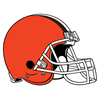Browns
2.0% implied probability

+5000
50 to 1Steelers
1.6% implied probability

+5000
50 to 1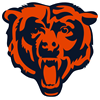Bears
1.6% implied probability

+6500
65 to 1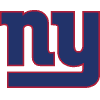Giants
1.2% implied probability

+6500
65 to 1Commanders
1.2% implied probability

+6500
65 to 1Patriots
1.2% implied probability

+6500
65 to 1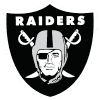Raiders
1.2% implied probability

+6500
65 to 1Rams
1.2% implied probability

+6500
65 to 1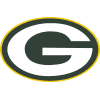Packers
1.2% implied probability

+7000
70 to 1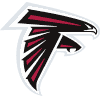Falcons
1.2% implied probability

+7500
75 to 1Panthers
1.1% implied probability

+7500
75 to 1Buccaneers
1.1% implied probability

+8000
80 to 1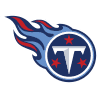Titans
1.0% implied probability

+10000
100 to 1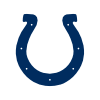Colts
0.8% implied probability

+20000
200 to 1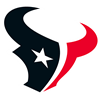Texans
0.4% implied probability

+20000
200 to 1Cardinals
0.4% implied probability

Latest NFL Articles
18 days ago
The defending NFC Champion Eagles will be back in the mix of things this season. How can you bet on the Eagles following the NFC schedule release?
20 days ago
With an expanded NFL holiday schedule this year, there will be plenty of NFL betting opportunities during the holiday season. Let's look at the matchups.
20 days ago
Some of the top games on this year's NFL schedule have been leaked online. What teams will be squaring off in their journey for the Lombardi Trophy?